# Apollonius and his ten problems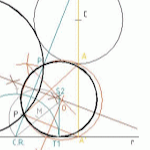One of the most comprehensive articles they have written my students in geometry classes is describing how to solve the so-called “Apollonius problems”.

Determining come straight circumferences or geometric constraints defined by the tangents are based on a family of geometric problems of great interest.

The group “AG-We are not hicks” introduces us rightly and thoroughness in this issue. Published initially aquí, belonging to the groups experience “Blogs experimental”, Article literally transcribe, adding some links in the text that complement. Thanks Diego, Alice, Clara, Sara and Sergio

## Apollonius and his ten problems

### Biography: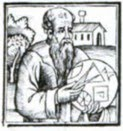Before developing the theories and Apollonius problem we will present a brief biography of Apolonio.

Greek mathematician Apollonius was born in Perge(262 B.C.- 190 B.C.),was a pupil of Archimedes. Neither is known about his life except for the introductions made in some of his treatises of which is composed his great work “The conicalused in the first terms: “ellipse, parabola and hyperbola“. He also discovered and described the “Epicycles” with which Ptolemy would use to explain the motion of planets. According to historians Apollonius had an irascible character which made him a difficult treatment.

Among the works of Apollonius of Perga geometric stand “The Places Planes” where operations are conducted most important things to know in the geometric design with a modern language and analytic geometry near as: dilation, translation, investment, rotation and similarity.

Information Obtained from the Book: “Technical Drawing” Antonio L.Blanco. “Wikipedia”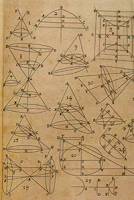One of the main contributions to the geometry of Apollonius is the proposed systemic problems tangencies, which are summarized in the following statement:

"Given three objects that can be, each one of them, points, the straight circles, dibujar one circle tangent to them three ".

The different problems tangencias derived from swap these elements give rise to them conocidos case study of the classical geometry, with the different Proposed Solutions that have been making it to the long history of.

Stand out 10 cases:

• three points,
• Three straight,
• points and one line,
• of straight lines and a point,
• points and one circle,
• of circles and a point,
• of straight lines and one circle,
• two circles and a line,
• point, a line and a circle
• three circles.

Another fundamental contributions of Apollonius, are The Conics.

Conic sections were known as Apollonius performed the study of these, but his treatise moves over the other theories. Previously it was believed that Apollonius the hyperbola, the parable, and ellipse sections were obtained from different cones according to the vertex angle.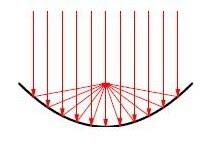So, Apollonius demonstrated that these curves can be obtained from the same sections of a cone, varying the inclination of the plane that intersects this. In addition to certifying that the cone does not have to be a right cone, may be circular, scalene or oblique.

Besides conic curves have interesting properties.

One of the most important Apollonius discovered are the reflection properties.

Reflection of the parable: if there is light from a distant source with a parabolic mirror, so that the incident rays are parallel to the axis of the mirror, then the light reflected by the mirror is focused on the focus.

Legend has it that Archimedes, contemporary of Apollonius, use this property to defend Syracuse from the Romans burned the ships of these. For this, produced a system of parabolic mirrors that concentrate sunlight got in the Roman ships.

Today the property has several utilities such as: radar systems, TV antennas or solar mirrors, inter alia.

Reflection of the ellipse: if a light source placed at the focus of an elliptical mirror, then the light reflected in the mirror is concentrated at the other focus.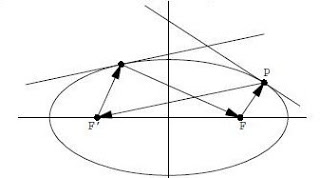Namely, if a ray from one focus, being reflected by the beam ellipse follow a path that went through the other focus.

Based on this property, we can see that if we have a pool table with elliptical, and launched the ball from one focus, with any direction, this bounce with game table and go through the other focus.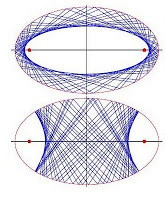If the ball bouncing would continue through the first focus, and so on, llagase until a time when the trajectory of the ball would be confused with the semi-major axis of the ellipse.

If instead they throw the ball from any point other than one focus was not one of the line that connects, segments of the ball trajectory figure describe another ellipse.

And conversely, if the starting point of the ball was a point on the line connecting the foci, this will draw the envelope of a hyperbola with the same foci.

Construction is curious elliptical ceiling rooms. When making a sound from one focus, this will sound with vivid clarity from the other focus. Also the sound will take the same time to spread from one focus to another regardless of the direction we take for broadcast. This effect also allows the soundproofing of rooms.

Reflection of the hyperbola: rays coming from one of the foci of a hyperbola are reflected so that the reflected rays appear to come from another source.

This property has been used for the creation of LORAN, which is a radio hyperbolic navigation device which has been used and is still used, Clearly, to a lesser extent due to the emergence of GPS and other systems, to fix the position of ships and aircraft.

Is based on the calculation of the time difference obtained in a receiver signals originating from the two transmitting stations located in the terrestrial surface.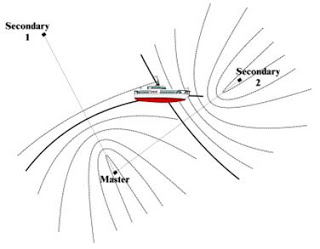As positioning is performed in two dimensions, if you know the difference of the distances to the two stations can locate the locus of points, where you can find the boat or plane, which is a hyperbola whose foci are the seasons.

Knowing the intersection of two or more hyperbolas is possible to define the position of the aircraft or ship.

## THE TEN PROBLEMS APOLONIO

Then we will treat 10 fundamental problems of Apollonius, which are based on the tangency between lines and circles.

Let's start talking about your main problem, from which solved all other cases, ie all ultimately be reduced to a circle that is tangent to another and passing through two points. Although his most difficult problem is to make a circle tangent to three.

### First and second problem

Previously this problem, there are simple to perform, which are: draw the circle through points portres(PPP) and draw the circle through two points and is tangent to a line(PPR). Are shown below:

### Third problem

Now let's focus on the case of a circle tangent to another and passing through two points. Steps to solve are as follows.

1. We find the bisector of the segment joining the given points, it must be the centers of the circles that we.
2. The line joining the points we know that will be the radical axis of the circles that we all.
3. Then draw an auxiliary circle passing through the points and cutting the given circle and draw a straight line connecting the points of intersection of the two circles. At the intersection of this line with the line connecting the two points (radical axis) found the radical center.
4. We find the tangents from the center to the circumference given radical, these points of tangency of the circles will also we are looking for.
5. Finally we add the points of tangency with the center of the circle and where the perpendicular cut the given points we get the solution circumferences centers.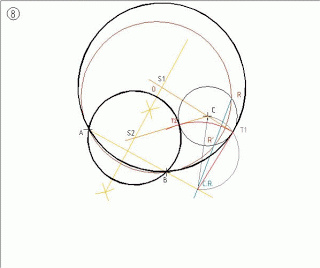### Fourth problem

Let's continue with the case of a circle tangent to three lines, in this case there will be four possible solutions, as will be shown below in the picture.

The procedure is simple:
-As we know the center of the circles must be in the internal and external bisectors that form three lines. Circumferences producing sought at the intersections of these lines.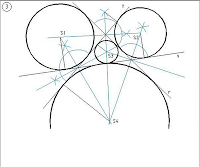### Fifth problem

The next case to explain a to be a circle tangent to two lines and which passes through a point.

In this case we speak of several possibilities: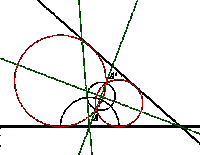1- If the lines are cut and the point is between them:

In this first case what we will hllar the bisector of the angle and find the counterpart of the given point, whereupon the problem is reduced to a circle tangent to a straight line that passes through two points

( explained above).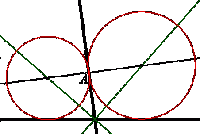2-: It may happen that the given point belongs to one of the given lines:

In the latter case we do is trace the bisectors of the two áangulos forming two lines and the given point draw a perpendicular to the line that contains it which bisectors wings cut in points sought, ie the centers of the circumferences.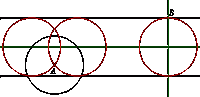3: Finally we will discuss the possibility that the two given lines are parallel.

A know that the point is between the two lines, therefore draw a circle with center A and diameter equal to the distance between the lines. Thus we obtain the centers of the two solutions at the intersection with the middle parallel. The point can also encontar in a given line such as point B , therefore find the center of the circle as the intersection of the solution average parallel and perpendicular to either of the two parallel lines at said point B.
Shown below:

### Sixth problem

This problem is based on making a circle tangent to two others and while passing through a point .. We will have four possible workarounds.

We consider the point that we give as a center for investment and taking one of the two circles, as self-inverting circumference, then plot the points dobles.Y circunferncia subsequently find the circunferenica of inversión.las circuanferencias tangents to figures given are inverses of the solution and also contain circumferences tangent points at its intersection with the dotted circunferncia find dobles.Posterioemente . Finally circumferences tarzar.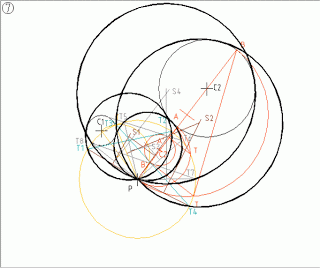### Seventh issue

We will explain how to perform the circunferncia tangent to two lines and that in turn is tangent to another circle dada.Podremos divide this problem into two:

1- Discuss the case where the given circumference is between the lines. The first step is to construct both sides of one of the straight lines parallel to a distance equal to the radius of the given circunferncia, Then find the symmetrical to the center of said circumference with respect to the bisetriz the angle formed by two lines. The straight line connecting the center and its short counterpart to one of the straight lines in a point, from that point we draw tangents to circunfercia center and passing through the center of the counterpart. Then draw an arc with center point and making found through the points of tangency, so what we get is cut parallel to the court first found at two points, finally got up from these points perpendicular to the bisector cut at two points, Serén which the centers of the circunferncias buscadas.Para to find the other two circunferncias solution all you have to do is repeat the process again with the other parallel, so we get the four solutions of the problem.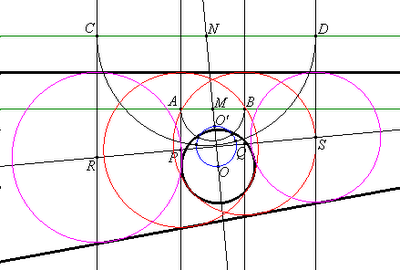2- It may happen that a given circumference tangent of the lines, therefore made to solve the same way as before, but two of the circles correspond to the pair solution external auxiliary ( is performed in the same way as before) and the other two solutions are reduced to a case where two lines intersect, since we know the nip one.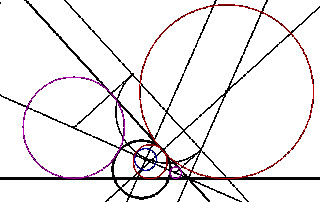### Eighth Problem:

In this case, the problem of Apollonius is given two circles and a line, find a circle that is tangent to the two circles and straight.

This complicated case, eight solutions, reduction is achieved by the case of a point (the center of one of the circumferences), a line (one parallel to the given) and a circumference (a concentric circle to the left). Concentric circumferences of the given circles have a radius R r and Rr being radii R and r given circumferences and parallel to the straight distance r is plotted in the given line.

So, these four circles were obtained considering a concentric circle of radius R r; of the four circumferences, two are obtained with one of the parallel and the other two with the other.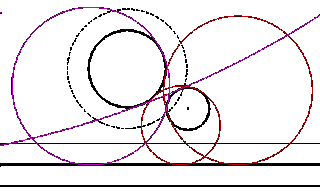These four circles are obtained solution now considering a concentric circle of radius Rr and again, one of the two parallel and two with the other.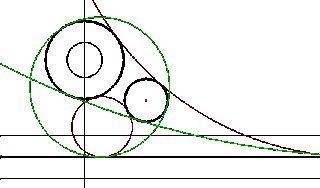Here's the eight solutions in the same figure.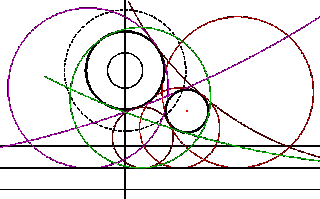### Ninth issue

Let DEVELOP the penultimate event of the ten problems of Apollonius before reaching the fundamental problem, in this case we will explain one circunerencia passing through a point and is tangent to a circle instead of a line.

Depending on the placement of the data we have four solutions but in some cases not reached any.

To relizarlo have to follow a series of steps:

1. The line is the investment figure the circumference , find a line perpendicular to that line and passing through the center of the given circunferecia, so we find the center of the circle investment( Point I in the drawing).
2. Arbitrary trace a circle passing through the given point and points that have straight cut tarzado the circumference and the counterpart straight dadas.Hallamos given point and also the radical and radical center axis.( points P and drawing P'en)
3. We trace the bisector between the points P and P 'and there you will find the centers of the circles solution. Then the arc able tarazamos 90 CR-O segment and thereby get to define the place of tangency T.
4. Focused on CR and CR-T cut radius r in T1 and T2. From T1 ar a perpendicular bisector cuts the PP’ in S2 and one from T2 cut perpendicular to S1, centers of the two circles solution.
5. Thus we obtain two solutions.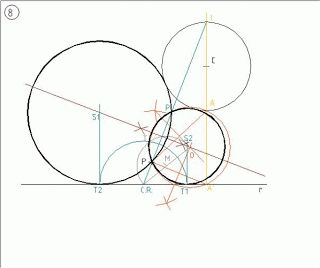1. In order to get the other two solutions we must consider the negative investment center and find A '. Arbitaria We drew a circle that passes through the points A, A 'and P and then as in the previous case we find the center point and axis P'y in radical.
2. We can arc segment 90 CR-O, thereby obtaining the location of tangency T as in the previous case cenro in CR and CR-T radius find the points of tangency 3 and 4 to cut the line in two points.
3. Draw the perpendicular bisector of the segment PP '. Since T3 a line perpendicular bisector cuts ar PP'en S3 and another perpendicular from T4 cut in S4, centers of the other two circles solution.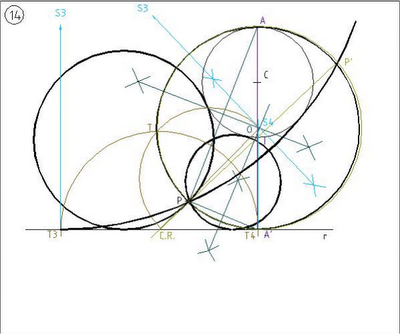### Tenth Problem.

Por último vamos a hablar del problema fudamental de Apolonio, in which a full circle tangent to three. En este caso podemos obtener hasta ocho soluciones dependiendo la forma en la que se encuentren las tres circunferencias que nos dan. Is performed as follows:

Lo primero que debemos hacer es hallar los seis centros de homotecia, three internal and three external, of the three circles that give us. These six points happen to be on four lines. Then what we do is take one of four straight and find the pole on the three circles, subsequently joined the radical center of the circle with three poles and get the points of tangency of the circles with circumferences dadas.Lo searched All we do now is to choose well among the six points of tangency found to draw two circles tangent. This procedure we have done with one of the straight, what we do with the other three in order to get the eight solutions.

It shows a picture of how it would be the final solution. It's a bit complicated performing this exercise and this is evident in this picture.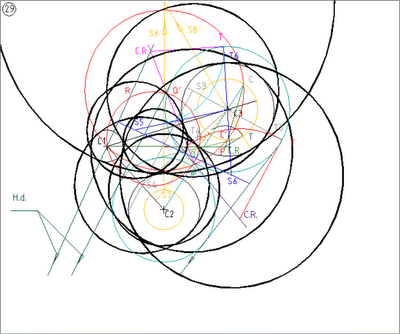Information obtained from: “Geothesis” “Zonabarbieri” and Bella geometry.